login  home  contents  what's new  discussion  bug reports help  links  subscribe  changes  refresh  edit

## A very brief introduction to programming in SPAD

• SPAD is an ordinary programming language, just like C, Java, and Python.
• The name SPAD comes from ScratchPad, which was the original name of the sytem FriCAS developed from.
• SPAD programs are ordinary text files that will be compiled to machine code by the SPAD compiler that comes with FriCAS.
• SPAD is a statically typed language with type levels.
1. Elements: These are the basic data objects. Examples are: 42, 3.14159265, "abc", [3,5,11]. They are what one usually considers as values in other programming languages.
2. Domains: These are the types of elements. For example, Integer is a type for 42, 3.14 is of type Float, "abc" is of type String, [1,2,4,8] is of type List(Integer).

Domains are comparable to classes in object oriented programming languages.

3. Categories: These are the types of domains. For example, Integer is of type IntegerNumberSystem, String is of type StringCategory, List(Integer) is of type ListAggregate(Integer).

Categories are somewhat comparable to interfaces in Java, but are much more powerful.

4. The highest type level is the constant keyword Category. In other words, categories like IntegerNumberSystem, StringCategory, ListAggregate(Integer) are of type Category.
• SPAD programs are usually definitions of categories and domains whose functionality will later be used in an interactive session or by other definitions.
• While categories define the exports of domains, i.e. which functions are provided, domains themselves implement the corresponding functions.
• Domains form a type hierarchy where only inheritance from one other domain is allowed.
• Categories form a type hierarchy with a multiple inheritance mechanism.
• Categories and domains are often called constructors and (as shown above) can be parametrized.
• SPAD has only very few built-in constructors. Most of the constructors are defined in a library. Builtin are Record, Tuple, Join, Mapping (abbreviated via ->). Library defined are Integer, List, String, Symbol, Monoid, Field, etc.

## A running example

Let us start with a little program. We do not to rely on any previously defined library, but we prefix every constructor with My in order to avoid name conflicts with existing names.

Our goal is to provide a domain MyFun that is parametrized by a domain S and represents functions from S into itself. We would like to be able to turn any function of type S -> S into an element of the MyFun(S) domain. Furthermore, we want to turn this domain into a monoid MyMonoid.

First we define the category MyMonoid.

fricas
(1) -> <spad>
fricas
)abbrev category MYMON MyMonoid
MyMonoid: Category == with
1: %
_*: (%, %) -> %</spad>
fricas
Compiling FriCAS source code from file
using old system compiler.
MYMON abbreviates category MyMonoid
------------------------------------------------------------------------
initializing NRLIB MYMON for MyMonoid
compiling into NRLIB MYMON
;;;     ***       |MyMonoid| REDEFINED
Time: 0.00 SEC.
finalizing NRLIB MYMON
Processing MyMonoid for Browser database:
--->-->MyMonoid(constructor): Not documented!!!!
--->-->MyMonoid(((One) (%) constant)): Not documented!!!!
--->-->MyMonoid((* (% % %))): Not documented!!!!
--->-->MyMonoid(): Missing Description
; compiling file "/var/aw/var/LatexWiki/MYMON.NRLIB/MYMON.lsp" (written 18 SEP 2023 05:09:29 AM):
; wrote /var/aw/var/LatexWiki/MYMON.NRLIB/MYMON.fasl
; compilation finished in 0:00:00.004
------------------------------------------------------------------------
MyMonoid is now explicitly exposed in frame initial
MyMonoid will be automatically loaded when needed from
/var/aw/var/LatexWiki/MYMON.NRLIB/MYMON

Every constructor needs an )abbrev line where one specifies whether the constructor to come is a category or domain. Then follows a capitalized identifier of at most 7 characters and finally the identifier for the constructor.

By convention, constructors begin with an uppercase letter and capitalize the first letter of each new word. Underscores are not commonly used.

Supposed the above code goes into a file mymonoid.spad, then this file can be compiled via:

    )compile mymonoid.spad


inside a FriCAS session.

Now comes the corresponding domain definition.

)abbrev domain MYFUN MyFun
MyFun(S: SetCategory): MyMonoid with
coerce:  (S -> S) -> %
coerce: % -> (S -> S)
elt: (%, S) -> S
Rep ==> S -> S
rep x ==> (x@%) pretend None pretend Rep
per x ==> (x@Rep) pretend %
coerce(f: S -> S): % == per f
coerce(x: %): S -> S == rep x
elt(x: %, s: S): S == (rep x) s
1: % == per((s: S): S +-> s)
((x: %) * (y: %)): % == per( (s: S): S +-> x y s )
   Compiling FriCAS source code from file
using old system compiler.
MYFUN abbreviates domain MyFun
------------------------------------------------------------------------
initializing NRLIB MYFUN for MyFun
compiling into NRLIB MYFUN
processing macro definition Rep ==> S -> S
processing macro definition rep x ==> pretend(pretend(@(x,%),None),S -> S)
processing macro definition per x ==> pretend(@(x,S -> S),%)
compiling exported coerce : S -> S -> %
MYFUN;coerce;M%;1 is replaced by f
Time: 0.00 SEC.
compiling exported coerce : % -> S -> S
MYFUN;coerce;%M;2 is replaced by x
Time: 0.00 SEC.
compiling exported elt : (%,S) -> S
Time: 0 SEC.
compiling exported One : () -> %
Time: 0 SEC.
compiling exported * : (%,%) -> %
Time: 0 SEC.
(time taken in buildFunctor:  0)
;;;     ***       |MyFun| REDEFINED
;;;     ***       |MyFun| REDEFINED
Time: 0 SEC.
Cumulative Statistics for Constructor MyFun
Time: 0.00 seconds
finalizing NRLIB MYFUN
Processing MyFun for Browser database:
--->-->MyFun(constructor): Not documented!!!!
--->-->MyFun((coerce (% (Mapping S S)))): Not documented!!!!
--->-->MyFun((coerce ((Mapping S S) %))): Not documented!!!!
--->-->MyFun((elt (S % S))): Not documented!!!!
--->-->MyFun(): Missing Description
; compiling file "/var/aw/var/LatexWiki/MYFUN.NRLIB/MYFUN.lsp" (written 18 SEP 2023 05:09:29 AM):
; wrote /var/aw/var/LatexWiki/MYFUN.NRLIB/MYFUN.fasl
; compilation finished in 0:00:00.008
------------------------------------------------------------------------
MyFun is now explicitly exposed in frame initial
MyFun will be automatically loaded when needed from
/var/aw/var/LatexWiki/MYFUN.NRLIB/MYFUN

This above code for MyFun can be in the same file as the code for MyMonoid, then one compilation would be enough. If, however, it is in another file myfun.spad, then a call to:

    )compile myfun.spad


inside a FriCAS session would be necessary.

Now we can use our little program. For that, we enter a FriCAS session and type the following.

fricas
Z ==> Integer
Type: Void
fricas
MZ ==> MyFun Z
Type: Void
fricas
inc(z: Z): Z == z+1
Function declaration inc : Integer -> Integer has been added to
workspace.
Type: Void
fricas
double(z: Z): Z == 2*z
Function declaration double : Integer -> Integer has been added to
workspace.
Type: Void
fricas
minc    := inc :: MZ
fricas
Compiling function inc with type Integer -> Integer
LISP output:
(#<FUNCTION |*1;inc;1;initial|>)
Type: MyFun?(Integer)
fricas
mdouble := double :: MZ
fricas
Compiling function double with type Integer -> Integer
LISP output:
(#<FUNCTION |*1;double;1;initial|>)
Type: MyFun?(Integer)
fricas
f := mdouble * minc;
Type: MyFun?(Integer)
fricas
g := minc * mdouble;
Type: MyFun?(Integer)
fricas
f 1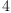(1)
Type: PositiveInteger?
fricas
g 1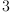(2)
Type: PositiveInteger?

Note that the multiplication is not commutative.

## Comments on the above program

• SPAD distinguishes between % and Rep. That's the reason for the definition of rep and per before MyFun. ((Note that the pretend None pretend Rep is only there because we are dealing with the domain S->S. In general pretend Rep is enough.))

The percent sign is a name for the current domain, it is comparable to this or self in other programming languages, but it does not denote the object, but rather its type, i.e., % stands for a domain.

In the definition of MyFun, % basically stands for MyFun(S). In contrast to that, Rep denotes the domain that the current domain inherits its data representation from (but not it's exports).

The distinction between % and Rep is in what they export. Whereas % exports all the functions that are listed in the category part of the domain, Rep points to a previously defined domain and thus exports exactly what is given there.

In our case Rep is the same as S -> S. Whereas % exports *, Rep does not. In contrast to that. Rep allows to write f(s) if f is of type S -> S and s is of type S, i.e. one can apply f to an argument of type S.

• The expression x y s stands for x(y(s)). In other words, juxtaposition in FriCAS associates to the right and usually means function application. Note, however, that x and y are of type % and not of type S -> S.

SPAD comes with a special feature. If in some context the compiler sees an expression a b with a of type A and b of type B, and there is a function

      elt: (A, B) -> C


then a b will be interpreted as elt(a, b).

In other words, the definition of elt: (%, S) -> S can be seen as syntactic sugar.

• Similar to Python, instead of braces, SPAD uses indentation to group code blocks.
• The escape character in SPAD is an underscore, not a backslash. Currently, the * identifier in the definition of MyMonoid must be escaped in that position. (There is hope that this need will go away in the future.)
• The symbol 1 in the definition of MyMonoid is not a number, but rather an identifier. Since in mathematics, 0 and 1 are used so often, both can be used as identifiers.
• One usually writes t: T to denote that t is of type T, i.e. with
      _*: (%, %) -> %


we declare that * is of type (%, %) -> %. The identifier -> is a builtin type constructor. Here it means that * is a function with two arguments, both of the same type, which returns a result of that type.

SPAD defines a few binary operators, like '+', '*', rem, quo to be infix. Except those few functions, all functions are used in prefix form, though.

• A category is defined by the following pattern
      C: Category == Join(C1,...,Cn) with
f1: T1
...
fk: Tk


where Join(...) can be missing or just be a single category C1.

• A domain is defined by the following pattern
      D: C == A add
Rep ==> A
rep x ==> (x@%) pretend Rep
per x ==> (x@Rep) pretend %
f1: T1 == ...
...
fk: Tk == ...


where C is a category and A is a domain from which D inherits.

If a domain A appears in front of the add keyword, then D inherits also all the implementations of the functions that are listed in the category part C.

       X ==> Y
macro X == Y


Both of the above lines are doing the same thing, they define a macro X that expands to Y whenever it appears elsewhere in the program code. Of course, only one of these lines would be sufficient.

Macros can have parameters.

• The notation
      (s: S): S +-> ....


is the SPAD way to denote lambda expressions (unnamed functions).

• The notation x @ X means x will be of type X. That is rarely seen in SPAD, but since SPAD not only allows to distinguish functions by their input types, but also their output types, it is sometimes necessary.

For example, in SPAD = is not builtin. It is an ordinary function of type

      (%, %) -> T


where T can be different things. For example, the domain Integer exports a function

      _=: (%, %) -> Boolean


with the usual meaning of equality. However, there is another domain in FriCAS, namely Equation(Integer) that exports a function

      _=: (Integer, Integer) -> %


Now, without @ it would be impossible to tell what the type of

      42 = 7


is. It could be Boolean or Equation(Integer). If the result should be of type Boolean, we write

      (42 = 7)@Boolean

• The use of pretend in t pretend X is very dangerous. It tells the compiler to consider t as an element of type X even though it might be of a type T with a completely different memory layout. In other words "abc" pretend Integer would interpret the storage of "abc" as an element of type Integer. Careless use of pretend usually leads to a program crash and should thus better be avoided.

Since % and Rep are supposed to have the same memory layout, pretend is safe in:

      rep x ==> (x@%) pretend Rep


Nevertheless is pretend a way to make the safety that SPAD brings with its type system void if it is not used with great care. In fact, pretend should be used only in these rare situations where the compiler is unable to figure out the right type itself.

• The notation t :: X is, in fact, equivalent to coerce(t)@X, i.e. a function with name coerce is called to turn the element t (which might be of type T) into an element of type X. In contrast to @ or pretend :: leads to the execution of this coercion at runtime.

See also How does one program in the AXIOM System . Note however, that this article is from 1992 actually describes the system AXIOM , i.e., the system that FriCAS forked from. Most of the text is still applicable. Nowadays instead of the dollar symbol, one has to use a percent sign to denote "current domain".

Since the Aldor programming language is very similar to SPAD, it might be advantageous to read the Aldor User Guide . There are, however, a number of differences between SPAD and Aldor . Nevertheless, it is possible to use the Aldor compiler to program new functionality for FriCAS.

You might want to try out Aldor .

To try out SPAD online you simply edit a wiki Sandbox page and put your code into \begin {spad} ... \end {spad} blocks.

## Making the example a bit more complex

The above code shows the basic way how to define categories and domains. Now we introduce inheritance and extend MyFun so that it becomes a structure that satisfies the Monoid type as defined in the FriCAS library.

Now (for demo purposes) we are going to prefix our new domains by ZZ in order to distinguish them from possibly existing names.

The FriCAS library already contains a definition of a Monoid . It's a bit richer than our MyMonoid from above, so we have to implement a few more functions in ZZFun. In particular, Monoid defines equality and output of elements.

Since we want to have coercions from and to this domain and also like to include function application directly, we start with a category that collects these functions.

)abbrev category ZZMON ZZMonoid
ZZMonoid(S: SetCategory): Category ==
Join(MyMonoid, CoercibleTo(S -> S), CoercibleFrom(S -> S)) with
elt: (%, S) -> S
)abbrev category ZZFMON ZZFunMonoid
ZZFunMonoid(S: Finite): Category == Join(ZZMonoid S, Monoid)
)abbrev domain ZZFUN ZZFun
ZZFun(S: SetCategory): ZZMonoid S with
if S has Finite then ZZFunMonoid(S)
Rep ==> MyFun S
rep x ==> (x@%) pretend Rep
per x ==> (x@Rep) pretend %
if S has Finite then
elements: List S := enumerate()$S ((x: %) = (y: %)): Boolean == for s in elements repeat if x s ~= y s then return false true coerce(x: %): OutputForm == of z ==> z::OutputForm pairs: List OutputForm := [paren [of s, of x s] for s in elements] bracket pairs spad  Compiling FriCAS source code from file /var/lib/zope2.10/instance/axiom-wiki/var/LatexWiki/1350013482118605993-25px004.spad using old system compiler. ZZMON abbreviates category ZZMonoid ------------------------------------------------------------------------ initializing NRLIB ZZMON for ZZMonoid compiling into NRLIB ZZMON ;;; *** |ZZMonoid| REDEFINED Time: 0 SEC. finalizing NRLIB ZZMON Processing ZZMonoid for Browser database: --->-->ZZMonoid(constructor): Not documented!!!! --->-->ZZMonoid((elt (S % S))): Not documented!!!! --->-->ZZMonoid(): Missing Description ; compiling file "/var/aw/var/LatexWiki/ZZMON.NRLIB/ZZMON.lsp" (written 18 SEP 2023 05:09:29 AM): ; wrote /var/aw/var/LatexWiki/ZZMON.NRLIB/ZZMON.fasl ; compilation finished in 0:00:00.000 ------------------------------------------------------------------------ ZZMonoid is now explicitly exposed in frame initial ZZMonoid will be automatically loaded when needed from /var/aw/var/LatexWiki/ZZMON.NRLIB/ZZMON ZZFMON abbreviates category ZZFunMonoid ------------------------------------------------------------------------ initializing NRLIB ZZFMON for ZZFunMonoid compiling into NRLIB ZZFMON ;;; *** |ZZFunMonoid| REDEFINED Time: 0.00 SEC. finalizing NRLIB ZZFMON Processing ZZFunMonoid for Browser database: --->-->ZZFunMonoid(): Missing Description ; compiling file "/var/aw/var/LatexWiki/ZZFMON.NRLIB/ZZFMON.lsp" (written 18 SEP 2023 05:09:29 AM): ; wrote /var/aw/var/LatexWiki/ZZFMON.NRLIB/ZZFMON.fasl ; compilation finished in 0:00:00.000 ------------------------------------------------------------------------ ZZFunMonoid is now explicitly exposed in frame initial ZZFunMonoid will be automatically loaded when needed from /var/aw/var/LatexWiki/ZZFMON.NRLIB/ZZFMON ZZFUN abbreviates domain ZZFun ------------------------------------------------------------------------ initializing NRLIB ZZFUN for ZZFun compiling into NRLIB ZZFUN processing macro definition Rep ==> MyFun S processing macro definition rep x ==> pretend(@(x,%),MyFun S) processing macro definition per x ==> pretend(@(x,MyFun S),%) ****** Domain: S already in scope augmenting S: (Finite) compiling exported = : (%,%) -> Boolean Time: 0.00 SEC. compiling exported coerce : % -> OutputForm processing macro definition of z ==> ::(z,OutputForm) Time: 0.00 SEC. ****** Domain: S already in scope augmenting S: (Finite) (time taken in buildFunctor: 0) ;;; *** |ZZFun| REDEFINED ;;; *** |ZZFun| REDEFINED Time: 0.00 SEC. Cumulative Statistics for Constructor ZZFun Time: 0.00 seconds finalizing NRLIB ZZFUN Processing ZZFun for Browser database: --->-->ZZFun(): Missing Description ; compiling file "/var/aw/var/LatexWiki/ZZFUN.NRLIB/ZZFUN.lsp" (written 18 SEP 2023 05:09:29 AM): ; wrote /var/aw/var/LatexWiki/ZZFUN.NRLIB/ZZFUN.fasl ; compilation finished in 0:00:00.008 ------------------------------------------------------------------------ ZZFun is now explicitly exposed in frame initial ZZFun will be automatically loaded when needed from /var/aw/var/LatexWiki/ZZFUN.NRLIB/ZZFUN ## Comments on the above program • Over an infinite domain S, we cannot algorithmically decide whether two functions from S into itself are equal or not. For finite S, however, algorithmic equality testing is possible. The same applies to printing. We, therefore, allow for arguments of ZZFunMonoid only finite domains. • The domain ZZFun(S), however, basically behaves like MyFun(S) if S is not finite. In fact, the line  == MyFun S add  says that ZZFun(S) inherits the implementation from MyFun(S). Everything that comes after the add keyword either overrides some functions from MyFun or implements new functionality. • If, however, the parameter S is finite, the number of exports is different. In other words, ZZFun(Integer) and ZZFun(PrimeField 5) have different exports. SPAD allows conditional exports as introduced via the line  if S has Finite then ZZFunMonoid(S)  for the category part (keyword with) and via the line  if S has Finite then  (and the following lines) for the implementation part (keyword add). • The notation  foo()$ Dom


means to call function foo from domain Dom.

It is important in two cases

1. if the functions from domain Dom have not been imported via
           import from Dom


and thus foo would not be in scope, or

2. if there are two functions foo with the same signature in scope, one from domain Dom and another from domain Baz. Then $Dom serves as disambiguator. • The symbol ~= means not equal and is defined in BasicType? as the negation of =. Equality testing and printing is not available for ZZFun(Integer). fricas ZZZ ==> ZZFun Z Type: Void fricas zz1 := inc :: ZZZ LISP output: (#<FUNCTION |*1;inc;1;initial|>) Type: ZZFun?(Integer) fricas zz2 := double :: ZZZ LISP output: (#<FUNCTION |*1;double;1;initial|>) Type: ZZFun?(Integer) fricas (zz1 = zz2)@Boolean There are 2 exposed and 10 unexposed library operations named = having 2 argument(s) but none was determined to be applicable. Use HyperDoc Browse, or issue )display op = to learn more about the available operations. Perhaps package-calling the operation or using coercions on the arguments will allow you to apply the operation. Cannot find a definition or applicable library operation named = with argument type(s) ZZFun(Integer) ZZFun(Integer) Perhaps you should use "@" to indicate the required return type, or "$" to specify which version of the function you need.
• However, it is available for ZZFun(PrimeField 5).

fricas
Z5 ==> PrimeField 5
Type: Void
fricas
ZZ5 ==> ZZFun Z5
Type: Void
fricas
inc5(z: Z5): Z5 == z+1
Function declaration inc5 : PrimeField(5) -> PrimeField(5) has been
added to workspace.
Type: Void
fricas
double5(z: Z5): Z5 == 2*z
Function declaration double5 : PrimeField(5) -> PrimeField(5) has
been added to workspace.
Type: Void
fricas
z51 := inc5 :: ZZ5
fricas
Compiling function inc5 with type PrimeField(5) -> PrimeField(5)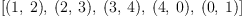(3)
Type: ZZFun?(PrimeField?(5))
fricas
z52 := double5 :: ZZ5
fricas
Compiling function double5 with type PrimeField(5) -> PrimeField(5)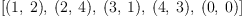(4)
Type: ZZFun?(PrimeField?(5))
fricas
(z51 = z52)@Boolean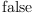(5)
Type: Boolean
• The domain OutputForm is used in the FriCAS interpreter to show elements. If a domain defines a function
      coerce: % -> OutputForm


then the interpreter knows how to show an element inside a FriCAS session.

Having a monoid, we can, of course, also use it to form a monoid ring.

fricas
P ==> MonoidRing(Z, ZZ5)
Type: Void
fricas
p1: P := 2*z51 - 1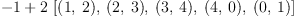(6)
Type: MonoidRing?(Integer,ZZFun?(PrimeField?(5)))
fricas
p2: P := 3*z52^3 + 2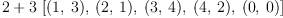(7)
Type: MonoidRing?(Integer,ZZFun?(PrimeField?(5)))
fricas
p1*p2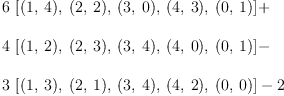(8)
Type: MonoidRing?(Integer,ZZFun?(PrimeField?(5)))

 Subject:   Be Bold !! ( 14 subscribers )